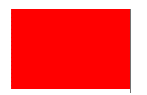# PLANE SHAPES

##### HELLO, WELCOME BACK TO CLASS

Plane shapes in mathematics are any closed, flat, 2-dimensional shapes. A plane shape has length and breadth, but no thickness. Plane shapes have properties including sides, corners, and faces.

Many plane shapes are polygons, or any 2-dimensional shape with straight sides or lines that is closed and has no open sides.  Polygons are plain figures or shapes made up of lines. Another type of plane shape is known as a quadrilateral, or a 2-dimensional shape with 4 straight sides that is closed and has no open sides.

## The Circle

The circle is a shape that can be made by tracing a curve that is always the same distance from a point that we call the center. The distance around a circle is called the circumference of the circle.

##The triangle is a shape that is formed by 3 straight lines that are called sides. There are different ways of classifying triangles, according to their sides or angles.

1. According to their angles:
• Right triangle: the largest of the 3 angles is a right angle.
• Acute Triangle: the largest of the 3 angles is an acute angle (less than 90 degrees).
• Obtuse Triangle: the largest of the 3 angles is an obtuse angle (more than 90 degrees).
1. According to their sides:
• Equilateral Triangle: all 3 sides are the same length.
• Isosceles Triangle: it has 2 (or more) sides that are of equal length. (An equilateral triangle is also isosceles.)
• Scalene Triangle: no 2 sides are of equal measure.

##The rectangle is a shape that has 4 sides. A rectangle has two equal sides of one length and two equal sides of a different length. A rectangle is like a stretched square. The distinguishing characteristic of a rectangle is that all 4 angles measure 90 degrees.

##A square has four sides, but not just any four sides. A square’s four sides are all the same length. A square with one-inch sides is smaller than a square with three-inch sides because one is less than three. A square also has four corners. The square is a type of rectangle, but also a type of rhombus. It has characteristics of both of these. That is to say, all 4 angles are right angles, and all 4 sides are equal in length.

##The trapezium also has 4 sides. It has two sides that are parallel but the other 2 are not.

##The rhombus is a shape formed by 4 straight lines. Its 4 sides measure the same length but, unlike the rectangle, any of all 4 angles measure 90 degrees.

The Parallelogram

A parallelogram is a quadrilateral with opposite sides parallel (and therefore opposite angles equal). A quadrilateral with equal sides is called a rhombus, and a parallelogram whose angles are all right angles is called a rectangle. A Parallelogram is a flat shape with opposite sides parallel and equal in length.

##How Can We Make ClassNotesNG Better? - CLICK to Tell Us Now!

Watch FREE Video Lessons for Best Grades on Afrilearn HERE!💃

### 3 thoughts on “PLANE SHAPES”

1.Pls I need mathematics questions and answers for primary four

2.3.